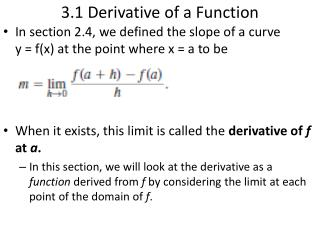DownloadDownload Presentation3.1 Derivative of a Function

# 3.1 Derivative of a Function

Télécharger la présentation## 3.1 Derivative of a Function

- - - - - - - - - - - - - - - - - - - - - - - - - - - E N D - - - - - - - - - - - - - - - - - - - - - - - - - - -
##### Presentation Transcript

1. 3.1 Derivative of a Function • In section 2.4, we defined the slope of a curve y = f(x) at the point where x = a to be • When it exists, this limit is called the derivative of f at a. • In this section, we will look at the derivative as a function derived from f by considering the limit at each point of the domain of f.

2. The domain of f ‘, the set of points in the domain of f for which the limit exists, may be smaller than the domain of f. If f ‘(x) exists, we say that fhas a derivative (is differentiable) at x. A function that is differentiable at every point of its domain is a differentiable function.

3. Applying the Definition • Differentiate (that is, find the derivative of) f(x) = x³.

4. After we find the derivative of f at a point x = a using the alternate form, we can find the derivative of f as a function by applying the resulting formula to an arbitrary x in the domain of f.

5. Applying the Alternate Definition • Differentiate using the alternate definition. • At the point x = a,

6. Notation • There are many ways to denote the derivative of a function y = f(x). Besides f’(x), the most common notations are these:

7. Relationships Between the Graphs of f and f’ • When we have the explicit formula for f(x), we can derive a formula for f’(x) using methods like those in examples 1 and 2. • The functions are encountered in other ways: graphically, for example, or in tables of data. • Because we can think of the derivative at a point in graphical terms as slope, we can get a good idea of what the graph of the function f’ looks like by estimating the slopes at various points along the graph of f.

8. Graphing f’ from f • Graph the derivative of the function f whose graph is shown in Figure 3.3a. Discuss the behavior of f in terms of the signs and values of f’.

9. Graphing f from f’ • Sketch the graph of a function f that has the following properties: 1. f(0) = 0 2. the graph of f’, the derivative of f, is as shown. 3. f is continuous for all x.

10. Solution • To satisfy property 1, begin with a point at the origin. • To satisfy property 2, consider what the graph of the derivative tells us about slopes. • To the left of x = 1, the graph of f has a constant slope of -1; therefore draw a line with slope -1 to the left of x = 1, making sure it goes through the origin. • To the right of x = 1, the graph of f has a constant slope of 2, so it must be a line with slope 2. There are infinitely many such lines, but only one – the one that meets the left side of the graph at (1 , -1) – will satisfy the continuity requirement.

11. Graphing the Derivative from Data • Discrete points plotted from sets of data do not yield a continuous curve, but we have seen that the shape and pattern of the graphed points (scatter plots) can be meaningful. • It is often possible to fit a curve to the points using regression techniques. • If the fit is good, we could use the curve to get a graph of the derivative visually.

12. One-Sided Derivatives • A function y = f(x) is differentiable on a closed interval [a , b] if it has a derivative at every interior point of the interval, and if the limits exist at the endpoints. • In the right-hand derivative, h is positive and a+h approaches a from the right. • In the left-hand derivative, h is negative and b+h approaches b from the left.

13. One-Sided Derivatives • Right-hand and left-hand derivatives may be defined at any point of a function’s domain. • The usual relationship between one-sided and two-sided limits holds for derivatives. • A function has a (two-sided) derivative at a point if and only if the function’s right-hand and left-hand derivatives are defined and equal at that point.

14. One-Sided Derivatives can Differ at a Point • Show that the following function has left-hand and right-hand derivatives at x = 0, but no derivative there.

15. Solution • We verify the existence of the left-hand derivative: • We verify the existence of the right-hand derivative: • Since the left-hand derivative equals zero and the right-hand derivative equals 2, the derivatives are not equal at x = 0. • The function does not have a derivative at 0.

16. Homework!!!!! • Textbook p. 105 # 1 – 17 ALL.